Get instant live expert help with Excel or Google Sheets“My Excelchat expert helped me in less than 20 minutes, saving me what would have been 5 hours of work!”

#### Post your problem and you’ll get expert help in seconds.

Your message must be at least 40 characters
Our professional experts are available now. Your privacy is guaranteed.

# Excel VLOOKUP Function Examples – VLOOKUP Tutorial, VLOOKUP Between Sheets

Excel’s VLOOKUP function provides you with a simple way to lookup and pull data from a range or table. There are many things you can do with VLOOKUP, including combining it with other functions. This is a list of the various VLOOKUP tutorials that you can reference on Excelchat to learn the function and help solve issues.

If you don’t find what you need on this list, you can always ask one of our Excelchat experts for some assistance through a live chat session.These tutorials will help you learn to use the VLOOKUP function in Excel.

## Learn How to Combine IF Function with VLOOKUP

This tutorial explains how the IF function is used with the VLOOKUP function to handle possible errors or tell Excel what to do “if” it finds what you’re looking for. The guide gives several examples and illustrations using IF and VLOOKUP together.

## How to Compare Two Columns in Google Sheets and Excel

This piece shows the reader how to locate duplicate or unique values when comparing two columns in Excel or Google Sheets. It uses the VLOOKUP, COUNTIF, MATCH, IF, and INDEX functions to accomplish this. There are several examples and illustrations.

## Check If One Column Value Exists in Another Column

Do you need to find out if the value in one column also exists in another column? If so, this tutorial not only provides a step-by-step guide with examples, but there is also a video tutorial included that walks you through the procedure.

## How to Apply Conditional Formal Based on VLOOKUP

This tutorial shows the reader how to use the VLOOKUP function to apply conditional formatting to cells in your Excel spreadsheet. It also shows how to copy conditional formats from one cell to another. There are several examples and illustrations to use as a guide.

## How to Use VLOOKUP Across Multiple Sheets

What happens when you want to use the VLOOKUP function across multiple sheets in Excel? This piece explains how this works and guides the reader through the process with several examples. It provides the basic syntax for the formula, which includes using the “consolidate” feature.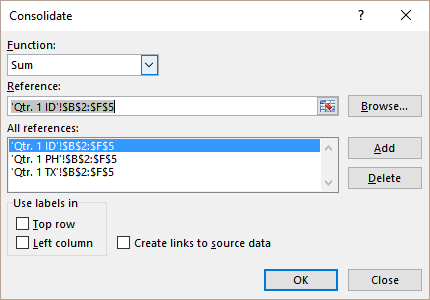Use the consolidate feature with VLOOKUP to work across multiple Excel sheets.

## Using VLOOKUP on Multiple Columns

VLOOKUP works by looking up items by “row” in Excel. This tutorial explains how you can use VLOOKUP to look through multiple columns for items that match either one or multiple criteria. There are several examples and illustrations in this piece.

## How to Lock a VLOOKUP from a Table Array

If you want to use multiple lookup values against a set of data, one way to do this is to lock the VLOOKUP from your table array. This piece explains how this works by providing the basic syntax to the formula and then walking through a detailed example with illustrations.

## Top Reasons Why Your VLOOKUP is Not Working, and How to Correct Them

If you’re trying to troubleshoot problems with the VLOOKUP function, this guide should help. It lists the most common reasons that the VLOOKUP function doesn’t work and shares solutions to these problems. There are detailed illustrations with each common issue.

## How to Use INDEX MATCH instead of VLOOKUP

When you’re trying to find certain values in Excel, there are several ways to accomplish this. If you don’t want to use VLOOKUP, and the alternative is to use INDEX MATCH. This guide explains how this compares to VLOOKUP and shows the reader how to use these functions with examples.

## VLOOKUP from Another Sheet

When working with large datasets, you might have more than one worksheet. This tutorial explains how VLOOKUP can still work between sheets in Excel. It provides examples of “looking up” data by specifying the name of the other sheet.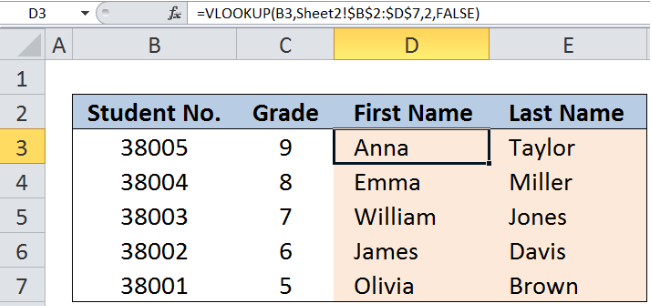You can learn how to use VLOOKUP between sheets in Excel.

## Handling an #N/A Error When Using VLOOKUP

As with any function in Excel, VLOOKUP can return an error when something isn’t right. This piece explains how you can deal with the #N/A error when it occurs with the VLOOKUP function. This generally occurs when the lookup value doesn’t exist, and the range is set to “false.”

## How to Copy a VLOOKUP Formula Down a Column

If you have a VLOOKUP formula that you want to use repeatedly down an entire column, this might not copy properly if it isn’t in the right format. This tutorial explains how to make sure you are using an absolute cell reference and then shows how to copy the formula.

## Learn How and When to Do a VLOOKUP in Google Sheets

VLOOKUP is just as effective for finding data in Google Sheets as it is in Excel. This piece explains how to use VLOOKUP in Google Sheets and provides a step-by-step guide for some of the most commonly used functions — including wildcards.

## Using VLOOKUP to Retrieve Information from Different Excel Files

VLOOKUP can also be used to get data from different Excel files. This guide shows how to accomplish this using the VLOOKUP and GETPIVOTDATA functions. It provides the syntax for the operation as well as a detailed example with illustrations.

## Nested VLOOKUP Formula Examples

VLOOKUP is a versatile function because it can be combined with a variety of other Excel functions to help you get the results you want. This tutorial shows the reader several nest VLOOKUP formula examples, meaning VLOOKUP is used several times or pulling from several different locations.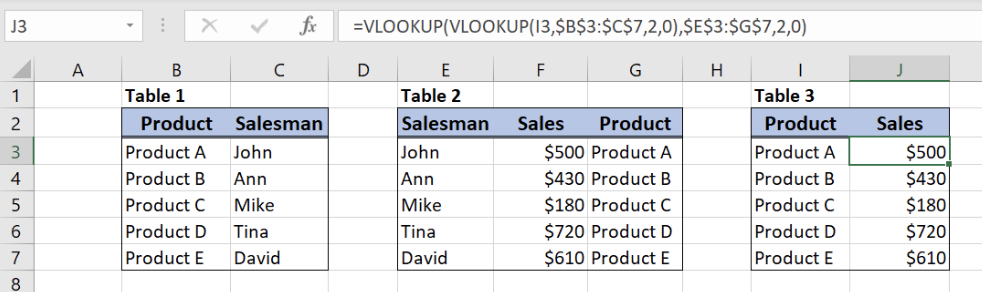Here are several examples of nested formulas with VLOOKUP.

## Lookup the Value Between Two Numbers

This guide shows the reader how to use the VLOOKUP function to locate a value that is between two numbers. The piece provides the basic syntax for the operation and then gives an example with illustrations.

## What Are Some Common Errors When Using VLOOKUP?

It can be frustrating to run into errors when using the VLOOKUP function. This is another tutorial that lists common errors associated with VLOOKUP and some possible solutions.

## What to Do If You’re Getting a #REF Error with VLOOKUP

If your VLOOKUP table is missing, Excel is probably going to return a #REF error with your VLOOKUP operation. This guide explains what to do if you get a #REF error with VLOOKUP.

## How to Pull Values from Another Worksheet in Excel

When using Excel, you might need an operation that pulls values from another worksheet. This guide explains how to use VLOOKUP to pull values from another sheet using a detailed example and illustrations.

## Excel Fuzzy Lookup

One of the other alternatives to using the VLOOKUP function is “fuzzy lookup.” This piece explains that fuzzy lookup is an Excel Add-In, shows where to access this, and explains its use as an alternative to VLOOKUP.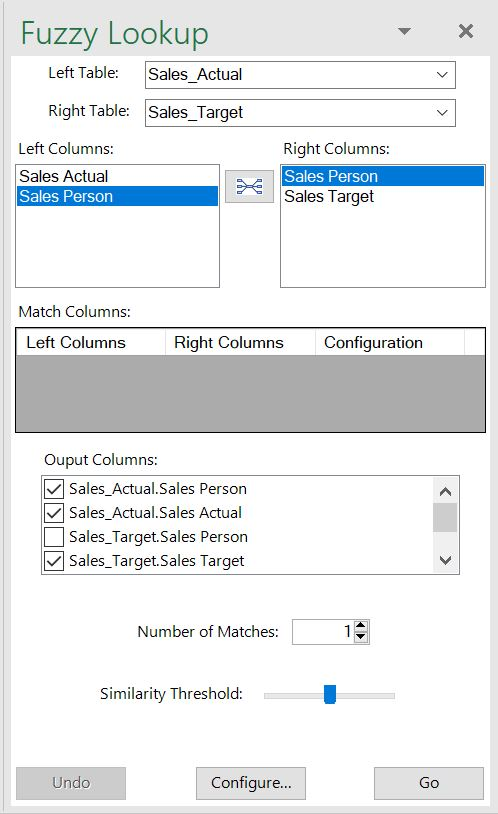Learn how to use the fuzzy lookup Add-In in Excel.

## How to Lookup with Variable Sheet Name

When the name of your worksheet varies in Excel, you can still use the VLOOKUP function to pull data when you combine it with the INDIRECT function. The piece gives the syntax for this operation and provides an example.

## How to Use VLOOKUP with Numbers and Text

This tutorial explains how you can use the VLOOKUP function to look for data in your Excel spreadsheet which is a mixture of both numbers and text. You will get the general formula to accomplish this as well as an example with illustrations.

## How to Use VLOOKUP by Date in Excel

Do you need to find certain dates within a large dataset in Excel? This piece shows the reader how to use the VLOOKUP function to locate data by data. It explains how to set up the range and gives the formula in an example using VLOOKUP.

## VLOOKUP with Multiple Criteria

When you use the VLOOKUP function, you give Excel criteria to use to search for your data. This tutorial explains how to use VLOOKUP when you have multiple criteria for your search instead of just one. It includes an example.

## VLOOKUP with Table Array: 5 Best Practices

This is a step-by-step guide on using the VLOOKUP function in Excel with a table array. It provides a series of best practices when working with this function alongside tables to pull specific data.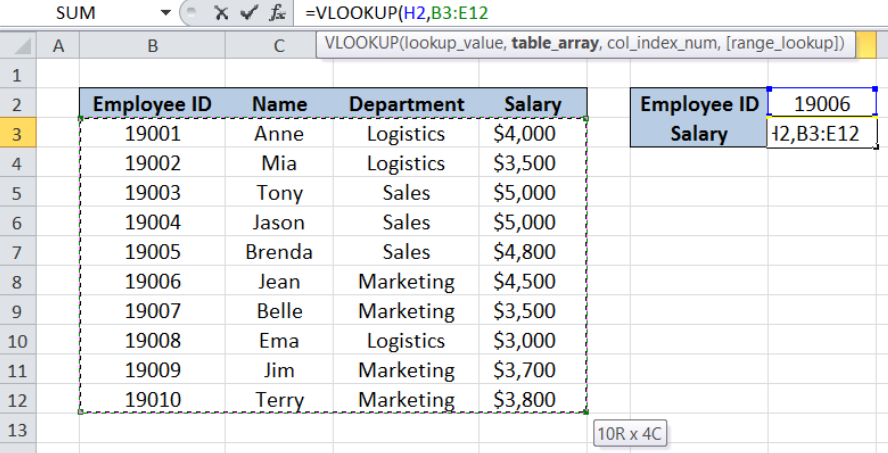Learn how to use VLOOKUP with a table array in Excel.

## How to Perform VLOOKUP Between Two Workbooks

This piece explains how the reader can use the VLOOKUP function to locate and extract data from two Excel workbooks instead of just one. To do this, you will need to use the INDIRECT, INDEX MATCH, and VLOOKUP functions together.

## Using VLOOKUP with Multiple Lookup Tables: Best Practices

This tutorial gives instruction on best practices for using VLOOKUP with multiple tables. Instead of just having a single range to search for data with the stated criteria, the reader will learn to specify several tables for the search with examples.

## Learn How to Calculate Shipping Cost with VLOOKUP

If you need your Excel spreadsheet to find data for shipping calculations, this piece shows how it can be accomplished using the VLOOKUP and MAX functions together. It illustrates how to set up the data and gives an example with the correct formula.

## How to Make VLOOKUP Reference Left Column in Excel

The VLOOKUP function finds a value in the leftmost column of a table. This piece explains how you can tell Excel to lookup a value even if it is not in the leftmost column by combining VLOOKUP with CHOOSE or using the INDEX MATCH functions.

## How to Combine the SUMIF and VLOOKUP Functions in Excel

This tutorial shows the reader how to combine the VLOOKUP and SUMIF functions in Excel in order to create a more dynamic SUMIF function. There are examples and illustrations provided as a guide.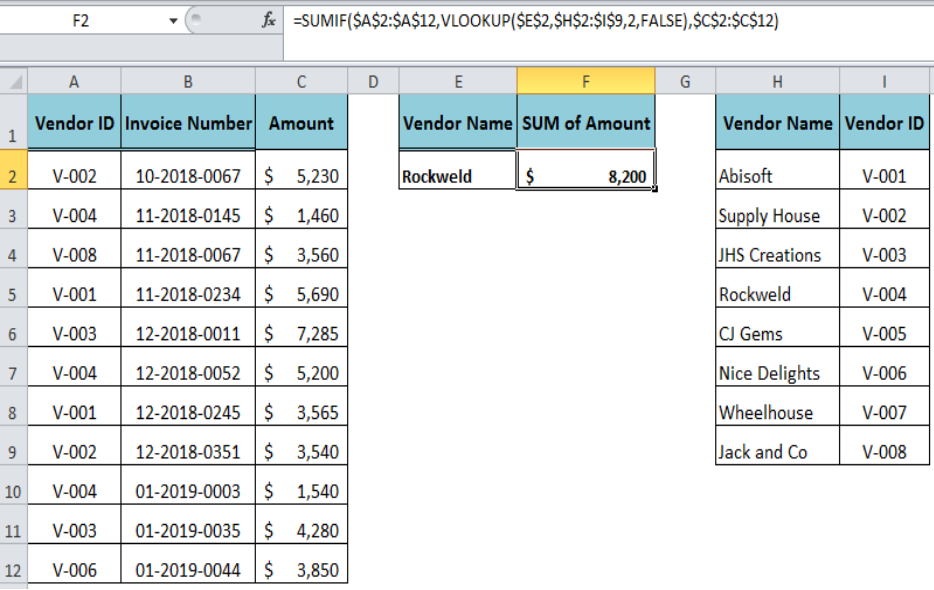Learn how to combine the VLOOKUP and SUMIF functions with this guide.

## Backwards VLOOKUP: A Quick Guide with Examples

If you need an alternative to VLOOKUP to do a reverse lookup, this piece explains how to do it by using the INDEX MATCH function. It shows the reader how to set up the data, gives the syntax for the operation, and provides an example.

## Example of VLOOKUP with the IF Condition

This piece explains how you can create a more dynamic VLOOKUP formula by combining it with the IF function. It provides an example of using IF to create another condition for the “lookup” of data in the Excel columns.

## How to Pull Data from Another Sheet in Google Sheets

VLOOKUP can be used in Google Sheets as well as in Excel. This tutorial shows how you can pull data from another sheet in Google Sheets using the VLOOKUP function. It provides an example with illustrations.

## Lookup Cost for Product or Service

This piece shows the reader how to lookup the cost of a product or service in an Excel spreadsheet using the VLOOKUP function. It provides an example with illustrations.

## VLOOKUP vs. HLOOKUP: When to Use Each Formula

This is an explanation of the difference between the VLOOKUP and HLOOKUP functions in Excel. It explains how each one is used, when to use each formula, and provides an example of each.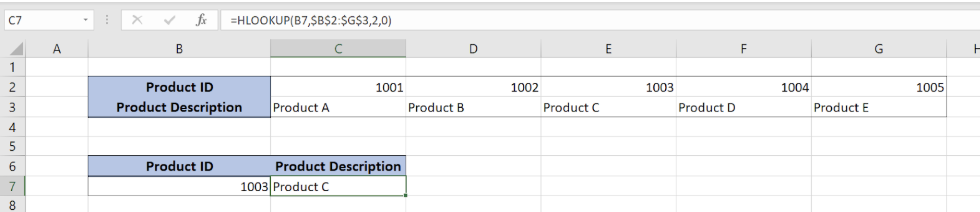Find out about the difference between VLOOKUP and HLOOKUP with this guide.

## VLOOKUP vs. LOOKUP: How Are They Different?

This tutorial explains the difference between the VLOOKUP and LOOKUP functions in Excel. It provides the syntax for both functions, explains their uses and limitations, and gives the reader examples of their use with illustrations.

## How to Get the nth Match with VLOOKUP in Excel

If you need to find the nth match in your Excel data, this piece shows how to accomplish this using the VLOOKUP function. It provides the formula for the operation as well as an example to use as a guide.

While this was a fairly exhaustive list of VLOOKUP tutorials, you still might find it lacking or may not want to sift through all of these different guides. Fortunately, there is another option.

Excelchat also offers one-on-one help that can either help you learn the VLOOKUP function or help you troubleshoot a problem-specific issue with Excel. Our friendly Excel experts are standing by 24/7 to lend a hand. Your first session is always free.